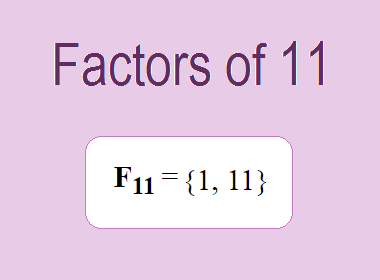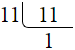# Factors of 11The factors of 11 are 1 and 11 i.e. F11 = {1, 11}. The factors of 11 are all the numbers that can divide 11 without leaving a remainder. 11 is a prime number, so it is divisible by 1 and 11 only.

We can check if these numbers are factors of 11 by dividing 11 by each of them. If the result is a whole number, then the number is a factor of 11. Let's do this for each of the numbers listed above:

·        1 is a factor of 11 because 11 divided by 1 is 11.

·        11 is a factor of 11 because 11 divided by 11 is 1.

## Properties of the Factors of 11

The factors of 11 have some interesting properties. One of the properties is that the sum of the factors of 11 is equal to 12. We can see this by adding all the factors of 11 together:

1 + 11 = 12

Another property of the factors of 11 is that the only prime factor of 11 is 11 itself.

********************

********************

## Applications of the Factors of 11

The factors of 11 have several applications in mathematics. One of the applications is in finding the highest common factor (HCF) of two or more numbers. The HCF is the largest factor that two or more numbers have in common. For example, to find the HCF of 11 and 22, we need to find the factors of both numbers and identify the largest factor they have in common. The factors of 11 are 1, and 11. The factors of 22 are 1, 2, 11, and 22. The largest factor that they have in common is 11. Therefore, the HCF of 11 and 22 is 11.

Another application of the factors of 11 is in prime factorization. Prime factorization is the process of expressing a number as the product of its prime factors. The prime factor of 11 is 11 since it is only the prime number that can divide 11 without leaving a remainder. Therefore, we can express 11 as:

11 = 11

We can do prime factorization by division method as given below,11 = 11

Since 11 is a prime number, there is no factor tree of 11.

## Conclusion

The factors of 11 are the numbers that can divide 11 without leaving a remainder. The factors of 11 are 1, and 11. The factors of 11 have some interesting properties, such as having a sum of 12. The factors of 11 have several applications in mathematics, such as finding the highest common factor and prime factorization.